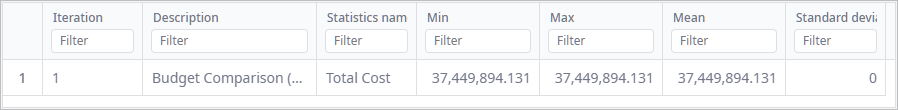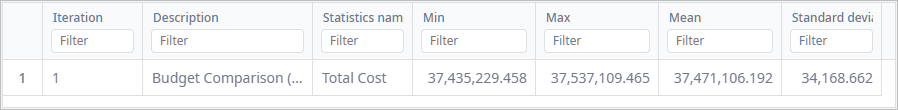anyLogistix

# Experiment Replication

Replication is a repeating run of a Simulation experiment. Replications are necessary for running experiments based on scenarios with stochastic parameters. If replications are not used, a single run of an experiment will not produce statistically significant results and will not allow for proper calculation of statistical data.

You can use replications when running Comparison, Variation, Safety stock estimation and Risk Analysis experiments.

Consider the following example. A Comparison experiment, which is based on a single scenario with stochastic parameters, runs twice: without replications and with them. The data for the Total cost statistic is collected during both runs, the minimum, maximum, mean and standard deviation values are calculated for this statistics.

In the first case, replications are not used. The Comparison experiment run therefore comprises a single run of the Simulation experiment. The single Total cost value is collected as a result of the single run. For this reason, the minimum, maximum and mean values are equal and the standard deviation value is zero:In the second case, replications are used and the Comparison experiment run comprises multiple runs of the Simulation experiment. The collected Total cost values might differ for each replication because the experiment scenario contains stochastic parameters. The minimum, maximum, mean and standard deviation values will be now properly calculated based on the collected statistics values:Replications are not necessary if the experiment scenario contains fixed parameters only. While you technically can perform several replications of such an experiment, you will get the same results for each replication.